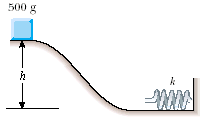Question# A 500 g block is released from rest and slides down africtionless track that begins

Other
ANSWEREDA 500 g block is released from rest and slides down africtionless track that begins h = 1.80 m above the horizontal, as shown in Figure. At the bottom of the track, where the surface ishorizontal, the block strikes and sticks to a light spring with aspring constant of 25.0 N/m. Find themaximum distance the spring is compressed.2020-11-17
Using conservationof energy since there is no friction in the process.
Whenthe block is at rest at height of h = 1.80m, the total energy isthe gravitational potential energy of the block mgh.
Whenthe spring is compressed to the maximum, the kinetic energy of theblock is 0
So $$\displaystyle{m}{g}{h}={0.5}{k}{x}^{{2}}$$
$$\displaystyle{x}={\frac{{{2}{m}{g}{h}}}{{{k}}}}$$
m =500g = 0.5kg,
g =9.8
h =1.80
k=25.0
so x =0.84 m
The spring can be maximum compresses 0.84 m